# CBSE Solutions for Class 10 English

#### GSEB std 10 science solution for Gujarati check Subject Chapters Wise::

Draw a line segment of length 6 cm. Using compasses and ruler, find a point P on it which divides it in the ratio 3 : 4. (2011D)

જવાબ :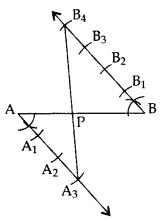=>  PA : PB = 3 : 4

Draw a line segment AB of length 7 cm. Using ruler and compasses, find a point P on AB such that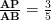. (2011OD)

જવાબ : AB = 7 cm, AB =… [Given in the question
AP : PB = 3 : 2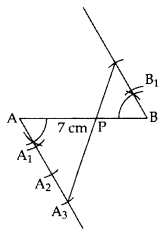AP : AB = 3 : 5 Constructions Class 10 Important Questions Short Answer-II (3 Marks)

Draw a triangle ABC in which AB = 5 cm, BC = 6 cm and ABC = 60°. Then construct a triangle whose sides aretimes the corresponding sides of ∆ABC. (2011D)

જવાબ : In ∆ABC
AB = 5 cm
BC = 6 cm
ABC = 60°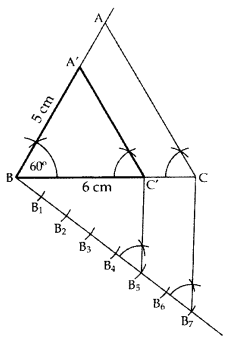∆A’BC’ is the required ∆.

Construct a triangle with sides 5 cm, 5.5 cm and 6.5 cm. Now construct another triangle, whose sides aretimes the corresponding sides of the given triangle. (20140D)

જવાબ :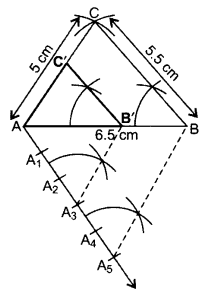∆AB’C’ is the required ∆.

Construct a right triangle in which the sides, (other than the hypotenuse) are of length 6 cm and 8 cm. Then construct another triangle, whose sides aretimes the corresponding sides of the given triangle. (2012D)

જવાબ : Here AB = 8 cm, BC = 6 cm and
Ratio =of corresponding sides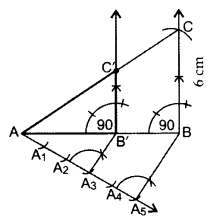∆AB’C’ is the required triangle.

Draw a triangle PQR such that PQ = 5 cm, P = 120° and PR = 6 cm. Construct another triangle whose sides aretimes the corresponding sides of ∆PQR. (2011D)

જવાબ : In ∆PQR,
PQ = 5 cm, PR = 6 cm,
P = 120°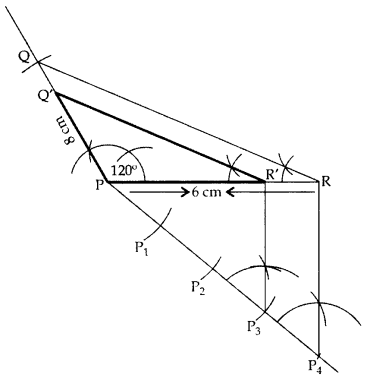∆POʻR’ is the required ∆.

Draw a triangle ABC with BC = 7 cm, B = 45° and C = 60°. Then construct another triangle, whose sides aretimes the corresponding sides of ∆ABC. (2012OD)

જવાબ : Here, BC = 7 cm, B = 45°, C = 60° and ratio istimes of corresponding sides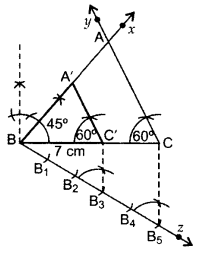∆A’BC’ is the required triangle.

Construct a triangle with sides 5 cm, 4 cm and 6 cm. Then construct another triangle whose sides aretimes the corresponding sides of first triangle. (2013D)

જવાબ :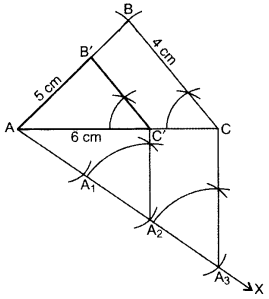Steps of Construction:

1. Draw ∆ABC with AC = 6 cm, AB = 5 cm, BC = 4 cm.
2. Draw ray AX making an acute angle with AÇ.
3. Locate 3 equal points A1, A2, A3 on AX.
4. Join CA3.
5. Join A2C’ || CA3.
6. From point C’ draw B’C’ || BC.
∆AB’C’ is the required triangle.

Draw a pair of tangents to a circle of radius 3 cm, which are inclined to each other at an angle of 60°. (2011OD)

જવાબ :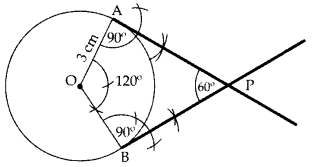PA & PB are the required tangents.

Draw a pair of tangents to a circle of radius 4.5 cm, which are inclined to each other at an angle of 45°. (2013OD)

જવાબ :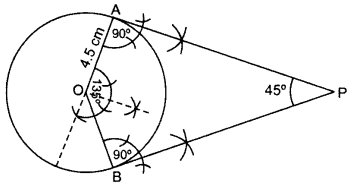Draw AOB = 135°, OAP = 90°, OBP = 90°
PA and PB are the required tangents.

Draw two tangents to a circle of radius 3.5 cm, from a point P at a distance of 6.2 cm from its centre. (2013OD)

જવાબ : OP = OC + CP = 3.5 + 2.7 = 6.2 cm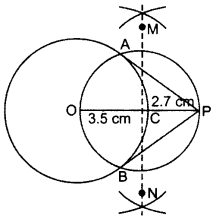AP & PB are the required tangents

Draw a right triangle ABC in which AB = 6 cm, BC = 8 cm and B = 90°. Draw BD perpendicular from B on AC and draw a circle passing through the points B, C and D. Construct tangents from A to this circle. (2014D, 2015OD)

જવાબ :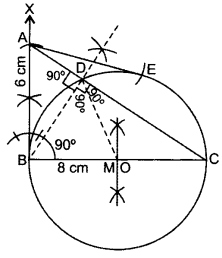Steps of Construction:

1. Draw BC = 8 cm.
2. From B draw an angle of 90°.
3. Draw an arc BA = 6 cm cutting the angle at A.
4. Join AC. ∆ABC is the required ∆.
5. Draw bisector of BC cutting BC at M.
6. Take Mas centre and BM as radius, draw a circle.
7. Take A as centre and AB as radius draw an arc cutting the circle at E. Join AE.
AB and AE are the required tangents.
Justification: ABC = 90° …[Given in the question
Since, OB is a radius of the circle.
AB is a tangent to the circle.
Also, AE is a tangent to the circle.

Find whether statement is True or False: - When a line segment is divided in the ratio 2/10, it is divided into 5 parts

જવાબ : True

Find whether statement is True or False: - When a line segment is divided in the ratio 2/10, it is divided into 1 parts

જવાબ : False

Find whether statement is True or False: - When a line segment is divided in the ratio 2/10, it is divided into 10 parts

જવાબ : False

Find whether statement is True or False: - When a line segment is divided in the ratio 2:10, it is divided into 5 parts

જવાબ : False

Find whether statement is True or False: - When a line segment is divided in the ratio 2:10, it is divided into 6 parts

જવાબ : True

Find whether statement is True or False: - When a line segment is divided in the ratio 2:10, it is divided into 2 parts

જવાબ : False

Find whether statement is True or False: - When a line segment is divided in the ratio 2:10, it is divided into 10 parts

જવાબ : False

Find whether statement is True or False: - The maximum number of common tangents that can be drawn to two circles intersecting at two distinct points is Infinte

જવાબ : False

Find whether statement is True or False: - When a line segment is divided in the ratio 2:10, it is divided into 1 parts

જવાબ : False

Find whether statement is True or False: - Tangent can be drawn without a circle

જવાબ : True

Find whether statement is True or False: - Tangent cannot be drawn without a circle

જવાબ : False

Find whether statement is True or False: - Angle between is radius and tangent is <90ᵒ

જવાબ : False

Find whether statement is True or False: - Angle between is radius and tangent is >90ᵒ

જવાબ : False

Find whether statement is True or False: - There are infinite tangent drawn from a circle.

જવાબ : True

Find whether statement is True or False: - There are finite tangent drawn from a circle

જવાબ : False

Find whether statement is True or False: - To divide a line segment PQ in the ratio 3:9, 3 equidistant point should be mark.

જવાબ : False

Find whether statement is True or False: - To divide a line segment PQ in the ratio 3:9, 2 equidistant point should be mark.

જવાબ : False

Find whether statement is True or False: - To divide a line segment PQ in the ratio 3:9, 9 equidistant point should be mark.

જવાબ : False

Find whether statement is True or False: - To divide a line segment PQ in the ratio 3:9, 12 equidistant point should be mark.

જવાબ : True

Find whether statement is True or False: - To divide a line segment PQ in the ratio 3/9, 3 equidistant point should be mark.

જવાબ : False

Find whether statement is True or False: - To divide a line segment PQ in the ratio 3:9, 2 equidistant point should be mark.

જવાબ : False

Find whether statement is True or False: - To divide a line segment PQ in the ratio 3:9, 9 equidistant point should be mark.

જવાબ : True

Find whether statement is True or False: - To divide a line segment PQ in the ratio 3:9, 12 equidistant point should be mark.

જવાબ : False

Find whether statement is True or False: - To divide a line segment PQ in the ratio 3:9, no equidistant point should be mark.

જવાબ : False

Find whether statement is True or False: - 1 tangent can be drawn from one point on the circle

જવાબ : True

Find whether statement is True or False: - 0 tangent can be drawn from one point on the circle

જવાબ : False

Find whether statement is True or False: - 2 tangent can be drawn from one point on the circle

જવાબ : False

Find whether statement is True or False: - Only 1 tangent can be drawn on a circle from a point outside the circle

જવાબ : False

Find whether statement is True or False: - Infinite tangent can be drawn from one point on the circle

જવાબ : False

Find whether statement is True or False: - 2 tangent can be drawn on a circle from a point outside the circle

જવાબ : True

Find whether statement is True or False: - No tangent can be drawn on a circle from a point outside the circle

જવાબ : False

Find whether statement is True or False: - Infinte tangent can be drawn on a circle from a point outside the circle

જવાબ : False

Find whether statement is True or False: - The maximum number of common tangents that can be drawn to two circles intersecting at two distinct points is 0

જવાબ : False

The maximum number of common tangents that can be drawn to two circles intersecting at two distinct points is 1

જવાબ : False

Find whether statement is True or False: - The maximum number of common tangents that can be drawn to two circles intersecting at two distinct points is 2

જવાબ : True

Find whether statement is True or False: - Angle between is radius and tangent is 90ᵒ

જવાબ : True

Draw a line segment AB of length 8 cm. Taking A as centre, draw a circle of radius 4 cm and taking B as centre, draw another circle of radius 3 cm. Construct tangents to each circle from the centre of the other circle. (2014OD)

જવાબ :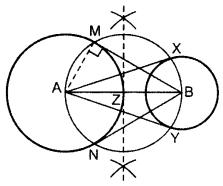Draw two circles on A and B as asked.
Z is the mid-point of AB.
From Z, draw a circle taking ZA = ZB as radius,
so that the circle intersects the bigger circle at M and N and smaller circle at X and Y.
Join AX and AY, BM and BN.
BM, BN are the reqd. tangents from external point B.
AX, AY are the reqd. tangents from external point A.

Construct an isosceles triangle whose base is 8 cm and altitude 4 cm and then another triangle whose side are 3/2 times the corresponding sides of the isosceles triangle.
Give the justification of the construction.

જવાબ :

Let us assume that ΔABC is an isosceles triangle having CA and CB of equal lengths, base AB of 8 cm, and AD is the altitude of 4 cm.

ΔAB'C' whose sides are 3/2 times of ΔABC can be drawn as follows.1. Draw a line segment AB of 8 cm. Draw arcs of same radius on both sides of the line segment while taking point A and B as its centre. Let these arcs intersect each other at O and O'. Join OO'. Let OO' intersect AB at D.
2. Taking D as centre, draw an arc of 4 cm radius which cuts the extended line segment OO' at point C. An isosceles ΔABC is formed, having CD (altitude) as 4 cm and AB (base) as 8 cm.
3. Draw a ray AX making an acute angle with line segment AB on the opposite side of vertex C.
4. Locate 3 points (as 3 is greater between 3 and 2) A1, A2, and A3 on AX such that AA1 = A1A2 = A2A3.
5. Join BA2 and draw a line through A3 parallel to BA2 to intersect extended line segment AB at point B'.
6. Draw a line through B' parallel to BC intersecting the extended line segment AC at C'. ΔAB'C' is the required triangle.

Justification

The construction can be justified by proving thatIn ΔABC and ΔAB'C',

ABC = AB'C' (Corresponding)

BAC = B'AC' (Common)

ΔABC ΔAB'C' (AA similarity criterion)… (A)

In ΔAA2B and ΔAA3B',

A2AB = A3AB' (Common)

AA2B = AA3B' (Corresponding angles)

ΔAA2B ΔAA3B' (AA similarity criterion)----(B)

On comparing equations (A) and (B), we obtainThis justifies the construction.

Draw the line segment AB = 5 cm. From the point A draw a line segment AD = 6cm making an angle of 600 with AB. Draw a perpendicular bisector of AD

જવાબ :Steps-

1. Draw the line segment AB = 5 cm
2. From the point A draw a line segment AD = 6cm
3. Make an angle of 600 on A with AB
4. Draw a perpendicular bisector of AD

A rhombus ABCD in which AB = 4cm and ABC = 60o, divides it into two triangles say, ABC and ADC. Construct the triangle AB’C’ similar to triangle ABC with scale factor 2/3.

જવાબ :Draw  ∆ABC in which BC = 7.5 cm, B =450 and AB – AC = 4

જવાબ :Steps:

1. Draw BC 7.5cm
2. Draw B =450
3. Cut an arc D 4cm on line of B and join CD
4. Draw perpendicular bisector on CD and name point A on intersection with BD
• ABC is the required ∆

Draw a circle with the help of a bangle. Take a point outside the circle. Construct the pair of tangents from this point to the circle.

જવાબ : Steps:

1. Draw a circle from a bangle.
2. Draw two non-parallel chords AB & CD
3. Draw the perpendicular bisector of AB & CD
4. Take the centre as O where the perpendicular bisector intersects.
5. To draw the tangents, take a point P outside the circle.
6. Join the points O & P.
7. Now draw the perpendicular bisector of the line PO and midpoint is taken as M.
8. Take M as centre and MO as radius draw a circle.
9. Let the circle intersects the circle at the points Q & R
10. Now join PQ & PR
11. Therefore, PQ & PR are the required tangentsConstruct a triangle with sides 5 cm, 6 cm and 7 cm and then another triangle whose sides are 7/5 of the corresponding sides of the first triangle.

જવાબ : Constructions:

1. Draw a line segment AB =5 cm.
2. Take A & B as centre, and draw the arcs of radius 6 cm & 5 cm respectively.
3. These arcs will intersect each other at point C, and therefore ΔABC is the required triangle with the length of sides as 5 cm, 6 cm, & 7 cm respectively.
4. Draw a ray AX which makes an acute angle with the line segment AB on the opposite side of vertex C.
5. Locate the 7 points such as A1, A2, A3, A4, A5, A6, A7 (as 7 is greater between 5and 7), on line AX such that it becomes AA1 = A1A2 = A2A3 = A3A4 = A4A5 = A5A6 = A6A7.
6. Join the points BA5 and draw a line from A7 to BA5 which is parallel to the line BA5 where it intersects the extended line segment AB at point B’.
7. Now, draw a line from B’ the extended line segment AC at C’ which is parallel to the line BC and it intersects to make a triangle.8.  ΔAB’C’ is the required triangle.

Draw a triangle ABC with side BC = 7 cm, B = 45° and ∆A = 105°. Then construct a triangle whose sides are 3/5 times the corresponding sides of ∆ABC. (2011D)

જવાબ : In ∆ABC, A + B + C = 180° … [angle sum property of a ∆
105° + 45° + C = 180°

C = 180° – 105° – 45o = 30°
BC = 7 cm∆A’BC’ is the required ∆.

Draw a triangle ABC with side BC = 6 cm, C = 30° and A = 105°. Then construct another triangle whose sides aretimes the corresponding sides of ∆ABC. (2012D)

જવાબ : BC = 6 cm, A = 105° and C = 30°In ∆ABC,
A + B + C = 180° …[Angle-sum-property
105° +
B + 30o = 180°
B = 180° – 105° – 30o = 45°
=>  ∆A’BC’ is the required ∆.

Draw a triangle with sides 5 cm, 6 cm and 7 cm. Then construct another triangle whose sides are 2/3 times the corresponding sides of the first triangle. (2012OD)

જવાબ : Given AB = 5 cm, BC = 7 cm, AC = 6 cm and ratio is  2/3 of corresponding sides.=>  ∆A’BC’ is the required triangle.

Construct an isosceles triangle whose base is 6 cm and altitude 4 cm. Then construct another triangle whose sides are ¾  times the corresponding sides of the isosceles triangle. (2015D)

જવાબ :=>  ∆A’BC’ is the required triangle.

Draw a line segment AB of length 7 cm. Taking A as centre, draw a circle of radius 3 cm and taking B as centre, draw another circle of radius 2 cm. Construct tangents to each circle from the centre of the other circle. (2015D)

જવાબ :Draw two circles on A and B.
Z is the mid-point of AB.
From Z, draw a circle taking ZA = ZB as radius,
such that the circle intersects the bigger circle at M & N and smaller circle at X & Y.
Join AX & AY, BM & BN.
BM, BN are the required tangents from external point B.
AX, AY are the required tangents from external point A.
Justification:

AMB = 90° …[Angle of a semi-circle

AM is a radius of the given circle.
=> BM is a tangent to the circle
Similarly, BN, AX and AY are also tangents.

Construct a ∆ABC in which AB = 6 cm, A = 30° and ∆B = 60°. Construct another ∆AB’C’ similar to ∆ABC with base AB’ = 8 cm. (2015OD)

જવાબ :Steps:

• Draw a ∆ABC with side AB = 6 cm, A = 30° and B = 60°.
• Draw a ray AX making an acute angle with AB on the opposite side of point C.
• Locate points A1, A2, A3 and A4 on AX.
• Join A3B. Draw a line through A4 parallel to A3B intersecting extended AB at B’.
• Draw a line parallel to BC intersecting ray AY at C’.
•  ∆AB’C’ is the required triangle.

Construct a triangle ABC in which AB = 5 cm, BC = 6 cm and ABC = 60°. Now construct another triangle whose sides are 5/7 times the corresponding sides of ∆ABC. (2015OD)
Solution:

જવાબ : In ∆ABC, AB = 5 cm; BC = 6 cm; ABC = 60°∆A’BC’ is the required ∆.

Construct a triangle ABC in which BC = 6 cm, AB = 5 cm and ABC = 60°. Then construct another triangle whose sides are ¾  times the corresponding sides of ∆ABC. (2016D)

જવાબ :Steps:

• Draw ∆ABC with the given data.
• Draw a ray BX downwards making an acute angle with BC.
• Locate 4 points B1, B2, B3, B4, on BX, such that BB1 = B1B2 = B2B3 = B3B4.
• Join CB4.
• From B3 draw a line C’B3 || CB4 intersecting BC at C’.
• From C’ draw A’C’ || AC intersecting AB at B’.
∆AB’C’ in the required triangle.

Draw a triangle ABC with BC = 7 cm, B = 45° and A = 105°. Then construct a triangle whose sides are 4/5 times the corresponding sides of ∆ABC. (2016D)

જવાબ : In ∆ABC, A + B + C = 180° ..[Angle-sum-property
105° + 45° +
C = 180°
C = 180° – 105° – 45o = 30°A’BC’ is the required triangle.

​​​​​​

Draw an isosceles ∆ABC in which BC = 5.5 cm and altitude AL = 3 cm. Then construct another triangle whose sides areof the corresponding sides of ∆ABC. (2016OD)

જવાબ :∆A’BC’ is the required ∆.

Draw a triangle with sides 5 cm, 6 cm and 7 cm. Then draw another triangle whose sides are 4/5 of the corresponding sides of first triangle. (2016OD)

જવાબ :∆A’BC’ is the required ∆.

Draw two concentric cirlces of radii 3 cm and 5 cm. Construct a tangent to smaller circle from a point on the larger circle. Also measure its length. (2016D)

જવાબ : Given, OD = 3 cm and OP = 5 cmPA and PB are the required tangents
By measurement PA = PB = 4 cm.

Draw a circle of radius 4 cm. Draw two tangents to the circle inclined at an angle of 60° to each other. (2013, 2016OD)

જવાબ : Draw a circle with O as centre and radius 4 cm.
Draw an
AOB = 120°. From A and B draw PAO = PBO = 90° which meets at P.PA and PB are the required tangents.

Draw a circle of radius 3 cm. Take two points P and Q on one of its extended diameter each at a distance of 7 cm from its centre. Draw tangents to the circle from these two points P and Q.

જવાબ : Steps:

1. Draw a circle with a radius of 3 cm with centre “O”.
2. Draw a diameter of a circle, and it extends 7 cm from the centre and mark it as P & Q.
3. Draw the perpendicular bisector of the line PO and mark the midpoint as M.
4. Draw a circle with M as centre and MO as the radius
5. Now join the points PA & PB in which the circle with radius MO intersects the circle of circle 3cm.
6. Now PA & PB are the required tangents.
7. Similarly, from the point Q, we can draw the tangents.
8. From that, QC & QD are the required tangents.### There are No Content Availble For this Chapter1 OC A 2.7cm 2 CP B 6.2cm 3 OP C 3.5cm 4 ∠OAP D 90ᵒ

જવાબ :

1-C, 2-A, 3-B, 4-D1 OA A 135ᵒ 2 ∠OAP B 4.5 cm 3 ∠AOB C 45ᵒ 4 ∠APB D 90ᵒ

જવાબ :

1-B, 2-D, 3-A, 4-C1 AP: BP A 7cm 2 ∠A B 2.8cm 3 BP C ∠B 4 AB D 3:2

જવાબ :

1-D, 2-C, 3-B, 4-A1 AP: BP A ∠A3PA 2 ∠A B 3:4 3 AA1 C ∠B 4 ∠B4PB D B1B

જવાબ :

1-B, 2-C, 3-D, 4-A1 BC:BC’ A 5:2 2 ∠B B 5cm 3 BC’:CC’ C 60ᵒ 4 AB D 7:5

જવાબ :

1-D, 2-C, 3-A, 4-B1 PR A ∠Q’ 2 ∠P B 6cm 3 ∠R C 120ᵒ 4 ∠Q D ∠R’

જવાબ :

1-B, 2-C, 3-D, 4-A1 AC A 3:5 2 BC B 6.5cm 3 AB’:AB C 5.5cm 4 AB D 5cm

જવાબ :

1-D, 2-C, 3-A, 4-B1 BC A 5:3 2 ∠B B 3.75cm 3 BC C ∠B’ 4 AB:AB’ D 6cm

જવાબ :

1-D, 2-C, 3-B, 4-A1 BC A 60ᵒ 2 ∠B B 7 cm 3 ∠C C 75ᵒ 4 ∠A D 45ᵒ

જવાબ :

1-B, 2-D, 3-A, 4-C1 AB A 4cm 2 BC B 6cm 3 AC C 5cm 4 AC:AC’ D 3:2

જવાબ :

1-C, 2-A, 3-B, 4-D

### Take a Test

Choose your Test :

### Constructions

Math
Chapter 11 : Constructions

### Browse & Download CBSE Books For Class 10 All Subjects

The GSEB Books for class 10 are designed as per the syllabus followed Gujarat Secondary and Higher Secondary Education Board provides key detailed, and a through solutions to all the questions relating to the GSEB textbooks.

The purpose is to provide help to the students with their homework, preparing for the examinations and personal learning. These books are very helpful for the preparation of examination.

For more details about the GSEB books for Class 10, you can access the PDF which is as in the above given links for the same.